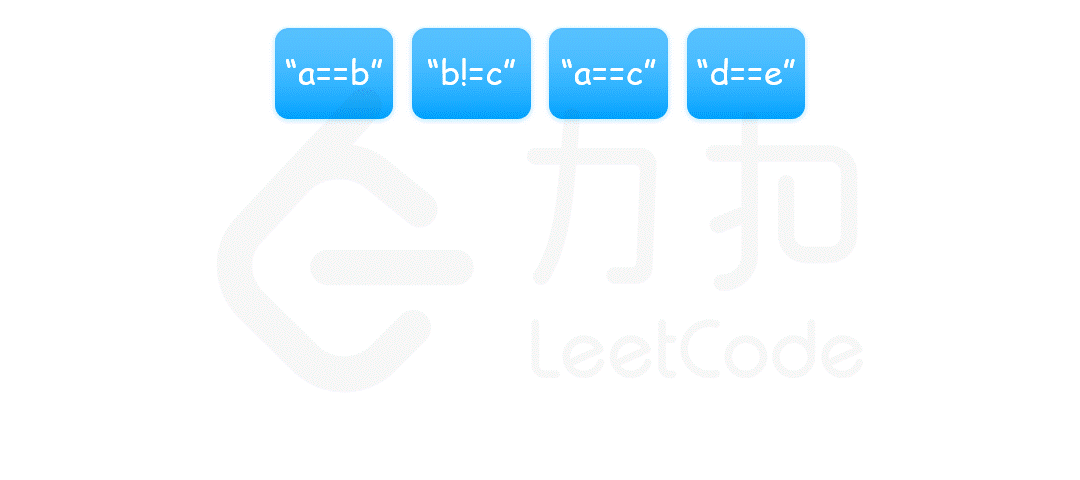## 练习## 990. 等式方程的可满足性

1 <= equations.length <= 500
equations[i].length == 4
equations[i] 和 equations[i] 是小写字母
equations[i] 要么是 '='，要么是 '!'
equations[i] 是 '='


### 方法class UnionFind {
private:
vector<int> parent;

public:
UnionFind() {
parent.resize(26);
iota(parent.begin(), parent.end(), 0);
}

int find(int index) {
if (index == parent[index]) {
return index;
}
parent[index] = find(parent[index]);
return parent[index];
}

void unite(int index1, int index2) {
parent[find(index1)] = find(index2);
}
};

class Solution {
public:
bool equationsPossible(vector<string>& equations) {
UnionFind uf;
for (const string& str: equations) {
if (str == '=') {
int index1 = str - 'a';
int index2 = str - 'a';
uf.unite(index1, index2);
}
}
for (const string& str: equations) {
if (str == '!') {
int index1 = str - 'a';
int index2 = str - 'a';
if (uf.find(index1) == uf.find(index2)) {
return false;
}
}
}
return true;
}
};C++提示：

C++中iota是用来批量递增赋值vector的元素

#include<iostream>
#include<numeric>       //iota头文件
using namespace std;
void func()
{
vector<int> v(10);
iota(v.begin(),v.end(),1);
vector<int>::iterator it = v.begin();
while(it != v.end())
{
cout<<*it++<<" ";
}
}

1 2 3 4 5 6 7 8 9 10